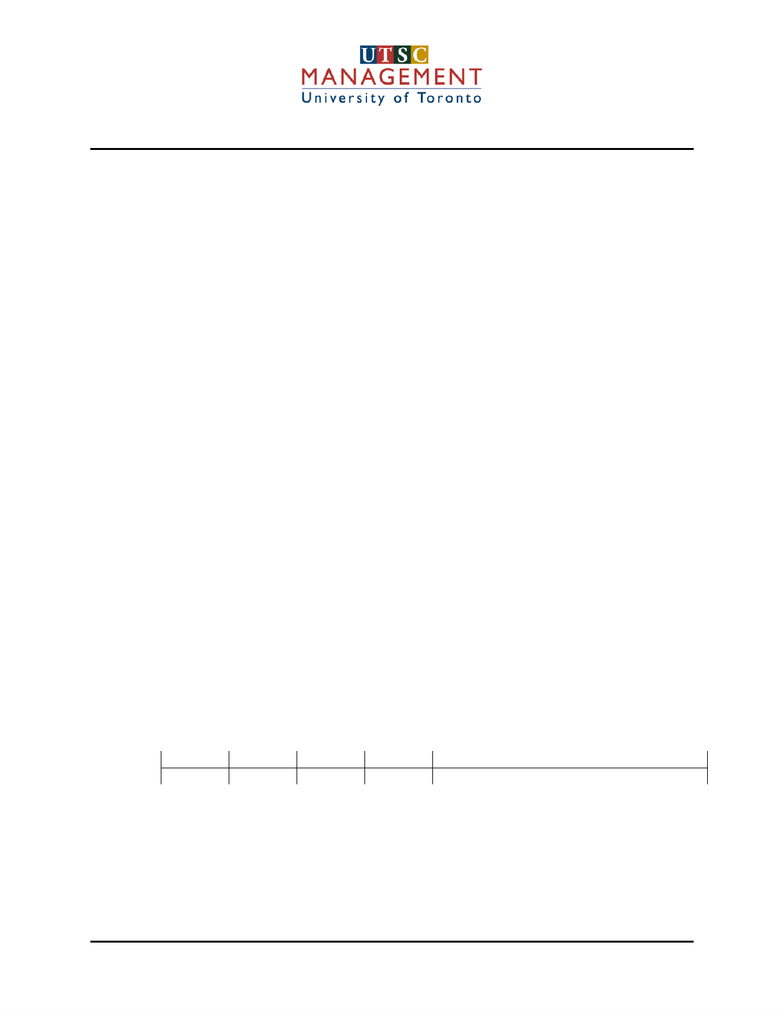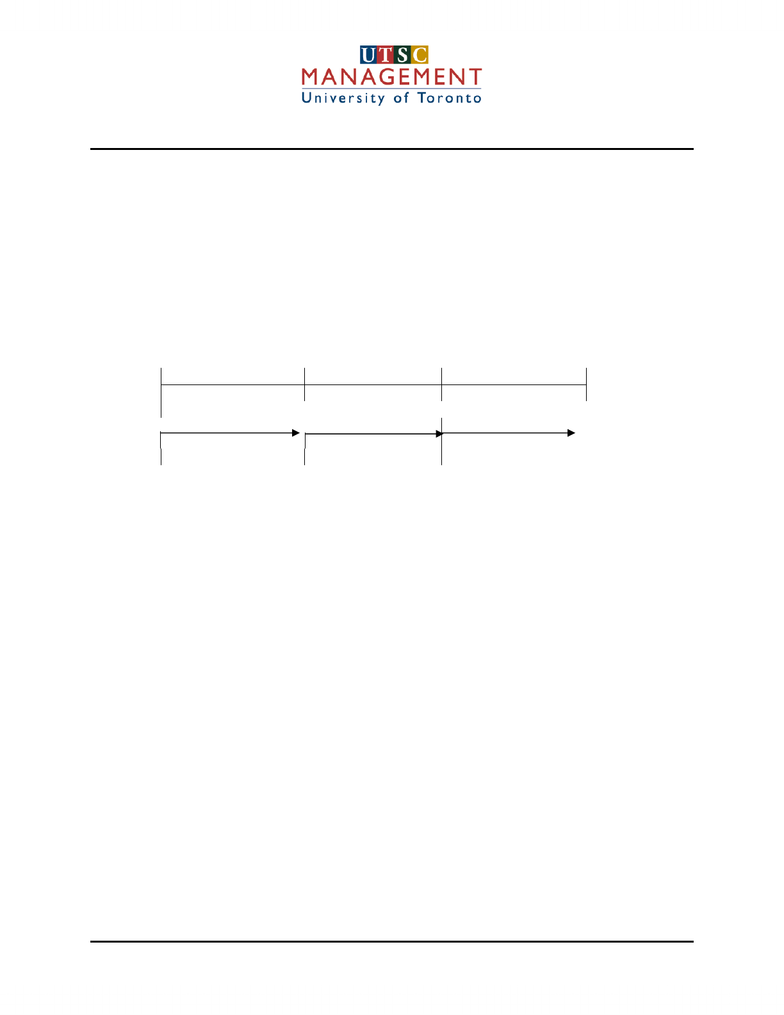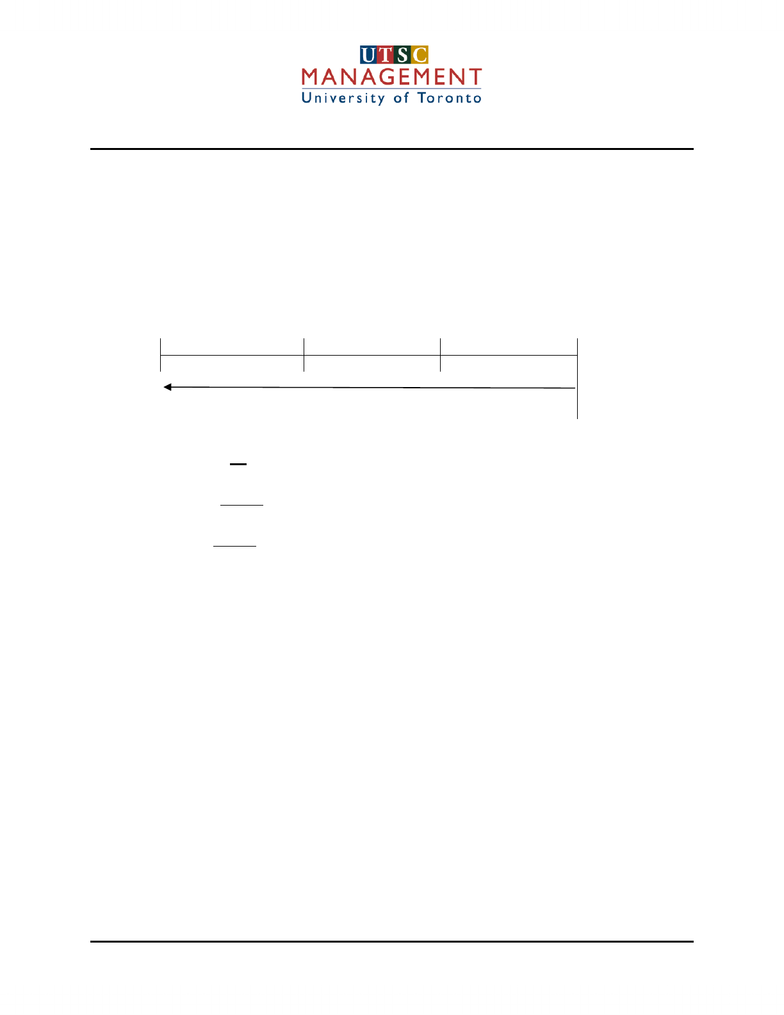Textbook Notes (290,000)
CA (170,000)
UTSC (20,000)
MGA (400)
Chapter

# Time Value of Money

Department
Financial Accounting
Course Code
MGAB02H3
Professor
Liang Chen

This preview shows pages 1-3. to view the full 16 pages of the document.Introductory Financial Accounting
Time Value of Money: Accounting & Business Applications
Prepared by Professor Sandra Daga Page 1 of 16
If someone were to ask you if you would rather have \$100 today or \$100 next year, your answer would be
“I’d like the \$100 today”. Your answer is based on the time value of money. The difference between the
worth of the two amounts is interest.
Many accounting and business applications use the time value of money. These applications include
accounting for bonds, leases, notes and pensions. Examples of business applications include what rate
of return is acceptable for investment and what interest rate to charge credit customers. There are
numerous personal applications as well such as the purchase of a home vs. renting, whether to borrow
for or lease a new car, and retirement planning.
Let’s say that you would like to do a little retirement planning and have decided you want to be a
millionaire by age 60. If you are 20 years old right now, and the after-tax rate of return is 1% per month,
how much would you have to save per month to achieve your goal? Only \$85 per month! That’s all! The
power of interest and time is on your side.
Simple & Compound Interest
Payment for the use of someone else’s money is called interest. There are two types of interest, simple
and compound. Generally, interest is specified in terms of a percentage rate for a period of time, usually a
year. For example, interest at 10% means the annual cost of borrowing an amount of money, called the
principal, is equal to 10% of that amount. If \$10,000 is borrowed for a period of one year at 10% annual
interest, the total to be repaid is \$11,000, \$10,000 principal and \$1,000 interest.
Most transactions, however, involve compound interest. Compound interest is the interest that accrues
on both the principal and the past unpaid accrued interest.
There are four basic types of compound interest computations:
1. Present value of a single sum due in the future.
2. Present value of an annuity, a series of receipts or payments.
3. Future value of a single sum at compound interest.
4. Future value of an annuity.
The easiest way to handle time value problems is to use a timeline to visualize the time periods and the
movement back and forth of money amounts. A timeline should be used for all but the very simplest
problems.
A timeline looks like this:
01345 n
Period
All receipts or payments should be placed on the time line and then the number of periods for movement
forward or back can be counted. Usually the present time is placed at period 0 but this can be adjusted
depending on the question.
Future Value

Only pages 1-3 are available for preview. Some parts have been intentionally blurred.Introductory Financial Accounting
Time Value of Money: Accounting & Business Applications
Future value is the amount to which a present given amount of money will grow, should interest be
charged or earned. The calculation of this amount will require the following information:
1. amount of the investment, which is the principal, “p”
2. rate of interest (rate of return), “i”
3. number of time periods, “n”
For example, we might want to determine the amount of money that we will have in our bank savings
account in 3 years should we deposit \$1,000 today, at an annual interest rate of 6%, if compounding is
annual.
The timeline looks like this:
0 1 2 3
\$1,000
Prepared by Professor Sandra Daga Page 2 of 16
\$1,000 x 1.06= \$1,060 \$1,060 x 1.06 = \$1,123.60 \$1,123.60 x 1.06=\$1191
The arithmetic determination would be as follows:
-end of year 1 we will have \$1000 + (\$1000 x 1.06) = \$1,060
-end of year 2 we will have \$1060 + (\$1060 x 1.06) = \$1,123.60
-end of year 3 we will have \$1123.60 +(\$1123.60 x 1.06) = \$1,191.00
The amount of \$1,191 is the future value of \$1,000 at 6% per year, compounded annually for 3 years.
This process can be shortened through the use of a formula as follows:
FV = P(1+i)n
For this example, using the formula:
FV = \$1,000 (1 + .06)3
= \$1,000 (1.191)
= \$1,191, which agrees to the arithmetic solution.

Only pages 1-3 are available for preview. Some parts have been intentionally blurred.Introductory Financial Accounting
Time Value of Money: Accounting & Business Applications
Present Value
The present value is the principal that must be invested at time period zero to produce the known future
amount.
Using the previous example, if a firm desires a return of 6% per year and is promised a future receipt of
\$1,191 in 3 years, then we can assume they will invest no more than \$1,000 today. The determination of
present value is a process of discounting, the reverse of the compounding calculations we performed to
find future value. We can then use the following timeline and formula:
The timeline is as follows:
0 1 2 3
? \$1,191
Present value (PV) = FV
(1 + i)n
= \$ 1,191
(1+.06)3
= \$ 1,191
1.191
= \$ 1,000
As you can see from the above, present value and future value are reciprocals of one another.
Compound Interest Tables
The present value formula noted need not be used, since tables are available that provide present value
factors.
Table 1 provides the amounts that must be deposited now at a stated rate of interest to equal \$1.00 at the
end of a stated number of periods.
Using Table 1 to solve for the present value for the example we have been using, we would refer to the
6% column, using the factor for 3 periods hence, which is (.84). The present value then would be
determined by multiplying the future value by the factor:
PV = \$1,191 x .84
= \$1,000 (rounded to the nearest dollar)
In our arithmetic solution of future value, we saw that at the end of year 2 we would have \$1,123.60.
Using Table 1 to find the present value of this amount, again we would refer to the 6% column, using the
factor for 2 years hence, (.89),
Prepared by Professor Sandra Daga Page 3 of 16